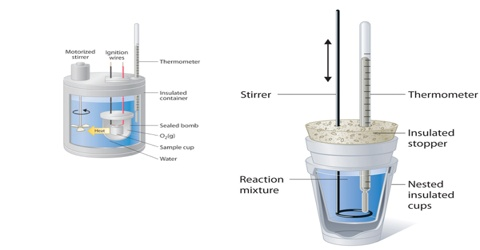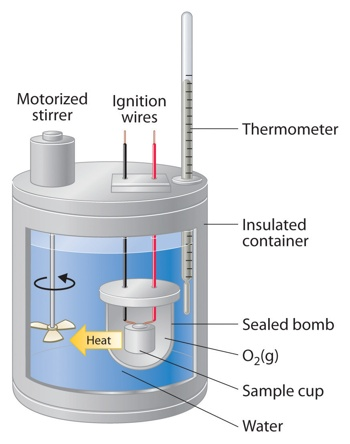# Calorimetry Determination of Heats of Reaction

Determination of Heats of Reaction: Calorimetry

Enthalpy changes in chemical reactions are usually measured with the help of calorimeters. A calorimeter is a simple device used for measuring the heat of chemical reactions or physical changes as well as heat capacity. In its simplest form, a calorimeter consists of a vessel or a beaker fitted with a thermometer and a stirrer. The temperature of the reaction mixture water is measured with a thermometer before and after the reaction. The total heat capacity of the calorimeter and water multiplied by the change in temperature gives a measure of the heat evolved or absorbed from which the enthalpy of reaction may be calculated. The temperature change is related to energy unit as;

Energy unit (kJ) = Specific heat capacity of water, (kJ kg-1K-1) x mass heated (kg) x change in temperature (K) = c x m x ∆t

This amount gives the absorbed or evolved for the quantity of reactants used in the experiment. Enthalpy change per mole of the reactant can be calculated from these data.

For good results the reaction should be rapid and should go to completion. The reaction mixture in the calorimeter should be stirred so that the change in temperature is uniform. Loss of heat due to radiation should be minimized as far as possible and a radiation correction introduced. The latter can be done by plotting the temperature of the calorimeter against time and extrapolating the curve to the time at which the reaction started. The corrected rise or fall in temperature is the difference between the extrapolated temperature and the initial temperature.

Adiabatic calorimetry makes the correction for loss due to radiation and conduction almost unnecessary. This method is particularly useful in measuring very small values of heats of reaction. Dewar flasks, i.e., vacuum walled vessels are used to minimize heat losses. In some types the outer jacket is electrically heated at a controlled rate such that the jacket is always kept at the same temperature as the calorimeter.Example: Calculate the amount of heat required to raise the temperature of 500 g of water by 5.0 K. The specific capacity of water is 4.18 J g-1K-1.

Solution: Heat Absorbed (q) = c x m x ∆T = 4.18 x 500 x 5.0 = 10,450 J.